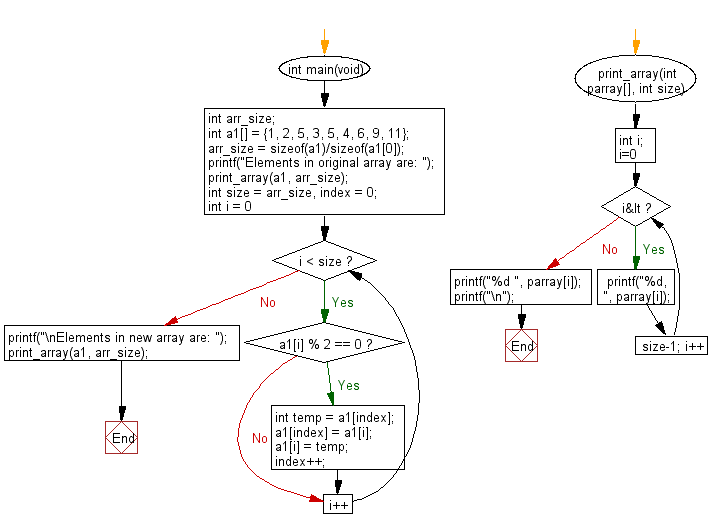﻿ C Program: Shift even numbers before odd in an array# C Exercises: Create new array from a given array of integers shifting all even numbers before all odd numbers

## C-programming basic algorithm: Exercise-73 with Solution

Write a C program to create new array from a given array of integers shifting all even numbers before all odd numbers.

C Code:

``````#include <stdio.h>
#include <stdlib.h>
int main(void){
int arr_size;
int a1[] = {1, 2, 5, 3, 5, 4, 6, 9, 11};
arr_size = sizeof(a1)/sizeof(a1);
printf("Elements in original array are: ");
print_array(a1, arr_size);

int size = arr_size, index = 0;
for (int i = 0; i < size; i++)
{
if (a1[i] % 2 == 0)
{
int temp = a1[index];
a1[index] = a1[i];
a1[i] = temp;

index++;
}
}
printf("\nElements in new array are: ");
print_array(a1, arr_size);
}

print_array(int parray[], int size)
{
int i;
for( i=0; i<size-1; i++)
{
printf("%d, ", parray[i]);
}
printf("%d ", parray[i]);
printf("\n");
}
``````

Sample Output:

```Elements in original array are: 1, 2, 5, 3, 5, 4, 6, 9, 11

Elements in new array are: 2, 4, 6, 3, 5, 1, 5, 9, 11
```

Pictorial Presentation:Flowchart:C Programming Code Editor:

What is the difficulty level of this exercise?

Test your Programming skills with w3resource's quiz.

﻿

## C Programming: Tips of the Day

Why doesn't a+++++b work?

printf("%d",a+++++b); is interpreted as (a++)++ + b according to the Maximal Munch Rule!.

++ (postfix) doesn't evaluate to an lvalue but it requires its operand to be an lvalue.

! 6.4/4 says the next preprocessing token is the longest sequence of characters that could constitute a preprocessing token"

Ref : https://bit.ly/3fdldUT